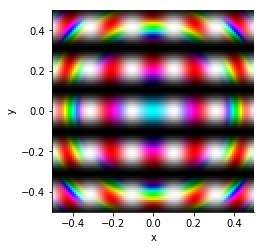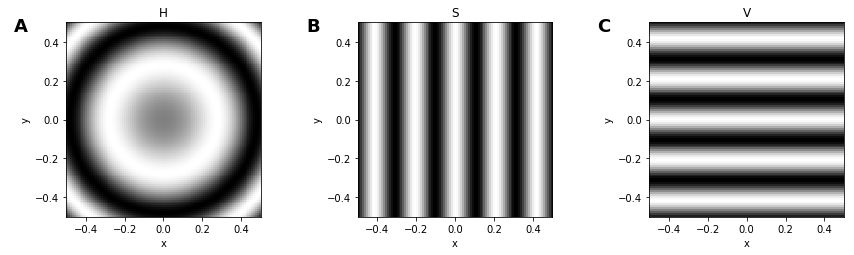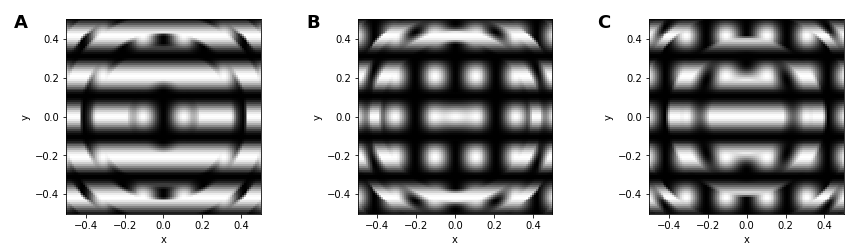# Hsv¶

Title
HSV Element
Dependencies
Matplotlib
Backends
Matplotlib
Bokeh
In :
import numpy as np
import holoviews as hv
from holoviews import opts

hv.extension('matplotlib')HSV represents a regularly spaced 2D grid of an underlying continuous space of HSV (hue, saturation and value) color space values. The definition of the grid closely matches the semantics of an Image or RGB element and in the simplest case the grid may be specified as a NxMx3 or NxMx4 array of values along with a bounds. An HSV element may also be defined through explicit and regularly spaced x/y-coordinate arrays. The two most basic supported constructors of an HSV element therefore include:

HSV((X, Y, H, S, V))



where X is a 1D array of shape M, Y is a 1D array of shape N and H/S/V are 2D array of shape NxM, or equivalently:

HSV(Z, bounds=(x0, y0, x1, y1))



where Z is a 3D array of stacked H/S/V arrays with shape NxMx3/4 and the bounds define the (left, bottom, right, top) edges of the four corners of the grid. Other gridded formats which support declaring of explicit x/y-coordinate arrays such as xarray are also supported. See the Gridded Datasets user guide for all the other accepted data formats.

In :
x,y = np.mgrid[-50:51, -50:51] * 0.1
h = 0.5 + np.sin(0.2*(x**2+y**2)) / 2.0
s = 0.5*np.cos(y*3)+0.5
v = 0.5*np.cos(x*3)+0.5

hsv = hv.HSV(np.dstack([h, s, v]))
hsv

Out:You can see how this is created from the original channels:

In :
opts.defaults(opts.Image(cmap='gray'))

hsv[..., 'H'].relabel('H') + hsv[..., 'S'].relabel('S') + hsv[..., 'V'].relabel('V')

Out:An HSV Element can also easily be converted to an RGB Element using the rgb property:

In :
print(hsv.rgb)
hsv.rgb[..., 'R'] + hsv.rgb[..., 'G'] + hsv.rgb[..., 'B']

:RGB   [x,y]   (R,G,B)

Out:For full documentation and the available style and plot options, use hv.help(hv.HSV).